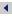Volatility is a popular measure of risk. It captures the fluctuations in the returns on the portfolio observed in the past.   Volatility is usually quoted as an annualized standard deviation by multiplying the monthly volatility and the square root of twelve. Monthly volatility is calculated as follows: monthly returns on the portfolio are observed and their mean is calculated; for each observation the difference to the mean is calculated and squared; the mean of these squares is called variance; its square root is generally called standard deviation and volatility in this context.   For a portfolio with a mean return of 10 percent and a volatility of 15 percent, the annual returns with a probability of 95 percent are likely to fluctuate in a band of two standard deviations about the mean, that is, between plus 40 and minus 20 percent.   Investors should keep an important lesson in mind: the future may always bring unexpected developments that cannot be forecasted from historical data.Back